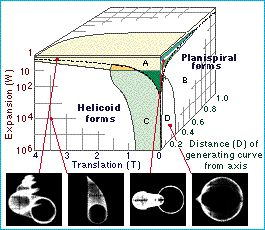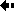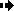MorphospaceA morphospace is a representation of the possible form, shape or structure of an organism. Each axis of the morphospace corresponds to a variable which describes some character of the organism. Each point in the morphospace represents an individual organism. This is illustrated in an animation.

Raup's analysis of shell shapes is an elegant example. Raup found that shell shapes could be described in terms of three main variables:

• translation rate,

• expansion rate,

• distance of generating curve from coiling axis.

As shown in the figure, any shell can be represented as a point in a three dimensional space, and Raup plotted the regions in this space that are occupied by living shells.

Figure: the three dimensional cube describes a set of possible shell shapes, four of which are illustrated; they were drawn on a computer. Only four regions in the cube (marked A, B, C, D) are actually occupied by natural species. All other regions in the cube represent theoretically possible but naturally unrealized shell shapes. From Raup (1966).Previous Next